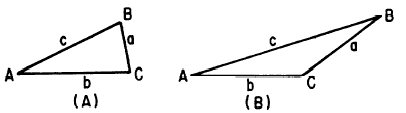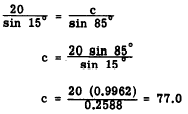Custom SearchLAW OF SINES The law of sines provides a direct approach to the solution of oblique triangles, avoiding the necessity of subdividing into right triangles. Let the triangle in figure 19-21 (A) represent any oblique triangle with all of its angles acute. The labels used in figure 19-21 are standardized. The small letter a is used for the side opposite angle A; small b is opposite angle B; small c is opposite angle C.Figure 19-20.-(A) Oblique triangle with all angles acute; (B) obtuse triangle.Figure 19-21.-(A) Acute oblique triangle with standard labels; (B) obtuse triangle with standard labels. The law of sines states that in any triangle, whether it is acute as in figure 19-21 (A) or obtuse as in figure 19-21 (B), the following is true:EXAMPLE: In figure 19-21 (A), let angle A-be 15° and let angle C be 85°. If BC is 20 units, find the length of AB. SOLUTION: By the law of sines,